# Class 12 Physics: CBSE Sample Question Paper- Term I (2021-22)- 4 Notes | Study Physics Class 12 - NEET

## NEET: Class 12 Physics: CBSE Sample Question Paper- Term I (2021-22)- 4 Notes | Study Physics Class 12 - NEET

The document Class 12 Physics: CBSE Sample Question Paper- Term I (2021-22)- 4 Notes | Study Physics Class 12 - NEET is a part of the NEET Course Physics Class 12.
All you need of NEET at this link: NEET

Class-XII
Time: 90 Minutes
Max. Marks: 35

General Instructions :

1. The Question Paper contains three sections.
2. Section A has 25 questions. Attempt any 20 questions.
3. Section B has 24 questions. Attempt any 20 questions.
4. Section C has 6 questions. Attempt any 5 questions.
5. All questions carry equal marks.
6. There is no negative marking.
Section - A

This section consists of 25 multiple choice questions with overall choice to attempt any 20 questions. In case more than desirable number of questions are attempted, ONLY first 20 will be considered for evaluation.

Q.1: An electric dipole has the magnitude of its charge as q and its dipole moment is p. It is placed in a uniform electric field E. If its dipole moment is along the direction of the field, the force on it and its potential energy are respectively :
(a) 2q⋅E and minimum
(b) q⋅E and p⋅E
(c) Zero and minimum
(d) q⋅E and maximum

From the question, as dipole moment is along direction of field, in such case angle between p and E zero, so the potential energy will be
U = − pE cos 0°
= − pE = minimum
It is observed that in uniform electric field, net force Fnet = 0

Q.2: If identical charges (−q) are placed at each corner of a cube of side b, then electric potential energy of charge (+q) which is placed at centre of the cube will be
(a) 8√2q2/4πε0b
(b) − 8√2q2/πε0b
(c) − 4√2q2/πε0b
(d) − 4q2/√3πε0b

Length of the diagonal of a cube having each side b is b√3 The distance of centre of cube from each vertex is √3/2b
As there are 8 vertices with charge (– q) at each corner, so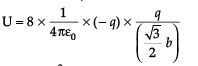U =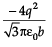Q.3: The electric intensity due to a dipole of length 10 cm and having a charge of 500 μC, at a point on the axis at a distance 20 cm from one of the charges in air, is:
(a) 6.25×107 N/C
(b) 9.28×107 N/C
(c) 13.1×1111 N/C
(d) 20.5×107 N/C

Considering electric field intensity
E = 9 × 109 × 2pr/(r2 − l2)2
Here, p = (500 × 106) × (10 × 10–2) = 5 × 10–5
Now, r = 25 cm = 0.25 m l = 5 cm = 0.05 m
Using the above expression and putting the values:
E = 9×10× 2 × 5×10−5 × 0.25/[(0.25)2−(0.05)2]2

= 6.25×107 N/C

Q.4: A point Q lies on the perpendicular bisector of an electrical dipole of dipole moment p. If the distance of Q from the dipole is r (much larger than the size of the dipole), then electric field at Q is proportional to :
(A) p−1 and r−2
(B) p and r−2
(C) p−1 and r−2
(D) p and r−3

In electric dipole, field
Eequatorial = kp/r3
E ∝ p, so E ∝ r−3

Q.5: A capacitor is charged by a battery. The battery is removed and another identical uncharged capacitor is connected in parallel. The total electrostatic energy of resulting system :
(a) decreases by a factor of 2
(b) remains the same
(c) increases by a factor of 2
(d) increases by a factor of 4

E = q2/2C
q’= q
C’ = 2C
E’ = E/2
Hence, total electrostatic energy of resulting system decreases by a factor of 2.

Q.6: A parallel plate air capacitor of capacitance C is connected to a cell of emf V and then disconnected from it. A dielectric slab of dielectric constant K, which can just fill the air gap of the capacitor, is now inserted in it. Which of the following is incorrect?
(a) The change in energy stored is 1/2CV2 (1/K – 1)
(b) The charge on the capacitor is not conserved
(c) The potential difference between the plates decreases K times.
(d) The energy stored in the capacitor decreases K times.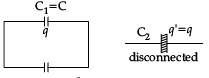q = CV ⇒ V = –q / C

• When a dielectric slab is inserted, new capacitance C2 = CK
• Initial energy stored in capacitor,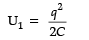• Final energy stored in capacitor,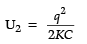Change in stored energy ΔU = U2 – U1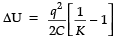=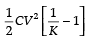• New potential difference between plates.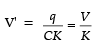• Charge on the capacitor is not conserved.

Q.7: A conducting sphere of radius R is given a charge Q. The electric potential and the electric field at the centre of the sphere respectively are :
(a) Zero and Q/4πεoR2
(b) Q/4πεoR and Zero
(c) Q/4πεoR and Q/4πεoR2
(d) Both are zero.

It is observed that in a conducting sphere, there is no charge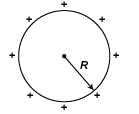Ein = Ecentre = 0
Vin = Vcentre = constant
= KQ/R = Q/4πεoR

Q.8: In the given figure, equivalent resistance between A and B will be :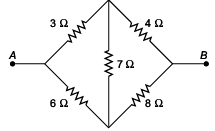(a) 14/3Ω
(b) 3/14Ω
(c) 9/14Ω
(d) 14/9Ω

In the Wheatstone bridge, the circuit is balanced as P/Q = R/S or 3/4 = 6/8.
If we re-draw the above circuit, we see that: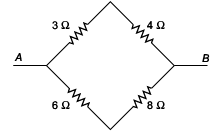Resistances 3 Ω and 4 Ω are in series, so the equivalent resistance is
3 Ω + 4 Ω = 7 Ω = R1
Also, resistances 6 Ω and 8 Ω are in series, so the equivalent resistance is
6 Ω + 8 Ω = 14 Ω = R2
Now after having equivalent resistances, if we further reduce the above circuit, we see that the equivalent resistances are in parallel.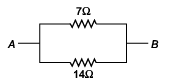So, Equivalent resistance is:
1/Rth = 1/R1 + 1/R2
1/Rth = 1/7 Ω + 1/14 Ω = 3/14 Ω
or Rth = 14/3 Ω

Q.9: An electron moving in a circular orbit of radius r makes n rotations per second. The magnetic field produced at the centre has magnitude :
(a) Zero
(b) μ0n2e/r
(c) μ0ne/2r
(d) μ0ne/2πr

Equivalent Current i = e/T
= e/(1/n) = en
Now Bc = μ0i/2r = μ0ne/2r

Q.10: Under the influence of a uniform magnetic field, a charged particle moves with constant speed V in a circle of radius R. The time period of rotation of the particle :
(a) depends on R and not on V
(b) is independent of both V and R
(c) depends on both V and R
(d) depends on V and not on R

Time period of rotation of the particle:
T = 2πm/qB, which is independent of V and R.

Q.11: A straight wire of diameter 0.5 mm carrying a current of 1A is replaced by another wire of 1 mm diameter carrying same current. The strength of magnetic field far away is :
(a) twice the earlier value
(b) same as earlier value
(c) one-half of the earlier value
(d) one-quarter of the earlier value

Magnetic field due to current carrying element is given by B=µ0i/2πr. Since, r is the distance between wire and measuring point.
Thus, the magnetic field B is independent of the diameter of wire. Hence, the magnetic field will be same.

Q.12: The average e.m.f. induced in a coil in which the current changes from 2A to 4A in 0.05 second is 8 volt. What is the self-inductance of the coil?
(a) 0.1 H
(b) 0.2 H
(c) 0.4 H
(d) 0.8 H

Induced emf, e = – L (di/dt)
As average e.m.f. induced in a coil is 8 V,
so 8 = L × (4 – 2)/0.05 or L = 0.2 H

Q.13: A step-up transformer operates on a 230 V line and supplies current of 2 A to a load. The ratio of the primary and secondary windings is 1 : 25. The current in the primary coil is :
(a) 15 A
(b) 50 A
(c) 25 A
(d) 12.5 A

Now IP/IS = Ns/Np
Ip = Is × (Ns/Np)
IP = 2 × 25 = 50 A

Q.14: An ac circuit consists of an inductor of inductance 0.5 H and a capacitor of capacitance 8 mF in series. The current in the circuit is maximum when the angular frequency of ac source is :

It is noted that current will be maximum at resonance, hence the resonant frequency will be: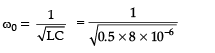Q.15: A cylindrical bar magnet of radius 0.8 cm is dropped through a metallic pipe of inner radius 1 cm. It takes more time to come down than it takes for a similar un-magnetized cylindrical iron bar dropped through the metallic pipe. This happens due to
(a) Friction
(b) Electrostatic charge developed on the inner wall of the pipe
(c) Generation of eddy current
(d) Buoyancy

When a cylindrical bar magnet is dropped inside a hollow cylindrical metallic pipe the magnetic flux across the pipe changes, and due to induction, eddy current is produced.

The direction of eddy current is in such a way so that it can oppose the cause, i.e., motion of magnet. So upward force acts on bar magnet and decreases the acceleration, which in turn takes more time to fall magnet through the pipe, as compared to simple metallic piece in place of magnet.

Q.16: Current in a circuit falls from 5.0 A to 0.0 A in 0.1 s. If an average emf of 200 V induced, the selfinductance of the circuit is
(a) 4 H
(b) 4 mH
(c) 40 mH
(d) 40 H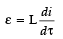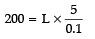L = 4H

Q.17: The polarity developed at the right end of 1st coil will be _______ pole and the polarity developed at the left end of the 2nd coil will be  _______ pole.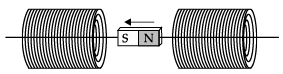(a) South, North
(b) North, South
(c) North, North
(d) South, South

Since the S-pole of the magnet is approaching the 1st coil, S-pole will be developed at the right end of the coil to oppose the movement of the magnet according to Lenz’s law.

Since the N-pole of the magnet is moving away from the 2nd coil, S-pole will be developed at the left end of the coil to oppose the movement of the magnet according to Lenz’s law.

Q.18: 1 Henry = volt-sec/ampere
(a) 10
(b) 1
(c) 103
(d) 10
–3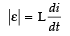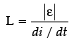Unit of L is Henry.
Unit of ε is volt.
Unit of di/dt is ampere / second.
∴ 1 Henry = 1 volt-sec/ampere

Q.19: The Q value of a series LCR circuit with L = 2.0 H, C = 32 μF and R = 10 Ω is
(a) 25
(b) 0.4 × 10–3
(d) 2500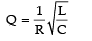=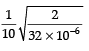= 25

Q.20: A device ‘X’ is connected to an a.c. source. The variation of voltage, current and power in one complete cycle is shown in Figure.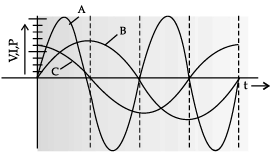Curve _______ shows power consumption over a full cycle and the device X is a _________ .
(a) A, capacitor
(b) A, inductor
(c) Either (A) or (B)
(d) B, capacitor

P = VI.

The amplitude of power is equal to multiplication of V and I. So it must be maximum amplitude as compared to V and I. So curve A shows the power consumption over a cycle.
The graph ‘A’ is symmetric with X-axis, i.e., area of graph on positive and negative sides are equal. So net area of power graph is zero. So power consumption in circuit is zero.
As the average power of device is zero, so phase difference between current and voltage is 900 and hence X is either an inductor or capacitor.

Q.21: A device X is connected across an ac source of voltage V = Vo sin wt. The current through X is given as I = Io sin (wt ± π/2 ). Which of the following graphs show the correct variation of voltage and current with time over one cycle of ac, for X.
(a)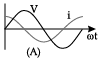(b)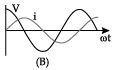(c)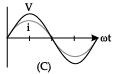(d)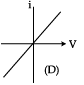Q.22: Which of the following transformer winding is correct?
(a)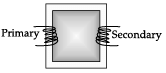(b)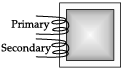(c)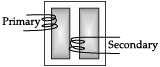(d)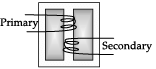In core type transformer, the primary and secondary winding are wound on both the limbs as shown in fig. (A).
In Shell type transformer, the winding are sandwiched on the central limb as shown in fig. (D)

Q.23: A silver wire has a resistance of 2.1Ω at 27.5°C, and a resistance of 2.7Ω at 100 °C. The temperature coefficient of resistivity of silver is
(a) 0.0039°C–1
(b) 0.039°C–1
(c) 0.39°C–1
(d) None of the above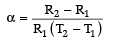=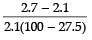= 0.0039°C–1

Q.24: Given the resistances of 1 Ω, 2 Ω, 3 Ω. How will these be combined to get an equivalent resistance of 11/3Ω?
(a) 1 Ω and 2 Ω in parallel and with this combination 3 Ω in series.
(b) 3 Ω and 2 Ω in parallel and with this combination 1 Ω in series.
(c) 1 Ω and 3 Ω in parallel and with this combination 2 Ω in series.
(d) all three resistors in parallel

When 1Ω and 2Ω are in parallel, the equivalent resistance is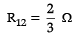When 3Ω and R12 are joined in series, the equivalent resistance is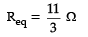Q.25: AB is a potentiometer wire (Figure). If the value of R is increased, balance point J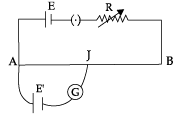(a) shift towards A
(b) shift towards B
(c) Remains in same position
(d) will be missing.

Section - B
This section consists of 24 multiple choice questions with overall choice to attempt any 20 questions. In case more than desirable number of questions are attempted, ONLY first 20 will be considered for evaluation.

Q.26: If distance between two charged particles is halved. How the force be affected?
(a) Half
(b) four times
(c) same
(d) twice

From Coulombs law, Force between two charged particles is inversely proportion to the square of distance between them.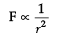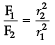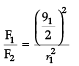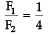⇒ F2 = 4F1
Therefore, force will increase to four times.

Q.27: Radius of the hydrogen atom is 10–15 m. The electric field at the surface of the atom is
(A) 14.4 × 10−20 NC−1
(B) 14.4 × 1020 NC−1
(C) 14.4 × 10−19 NC−1
(D) 14.4 × 1019 NC
−1

Electric field is given by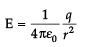Here, q = 1.6 x10-19C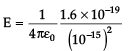=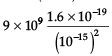= 14.4 × 1020 NC−1

Q.28: 8 small drops of equal size having radius of 2 mm are charged to 220 volt. Together they make a bigger drop. Calculate the potential of bigger drop.
(a) 220 V
(b) 1760 V
(c) 880 V
(d) 27.5 V

Let r be the radius of smaller drop and R be the radius of bigger drop.
Since volume of 8 smaller drops = volume of a bigger drop.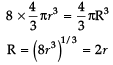= 4mm
As the capacity of bigger drop = 4 πε0R =4 πε02r
As potential on bigger drop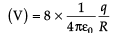=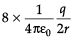=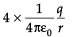= 4 x V
= 4 x 220
=880 Volt

Q.29: The maximum capacitance of three identical capacitors is obtained by connecting them
(a) in parallel
(b) in series
(c) two in parallel and one in series
(d) two in series and one in parallel

Maximum capacitance of capacitors is obtained when they are connected in parallel.

Cp= C1 + C2 + C3

when,C1 = C2 = C3
Cp= 3C
When these capacitors are connected in series, the effective capacitance is minimum.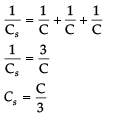When two in parallel and one in series, the effective capacitance is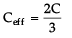When two in series and one in parallel, the effective capacitance is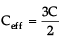Therefore, any combination of parallel and series capacitors, Ceff always be less them Cp.

Q.30: A current of 8.0 ampere is flowing in a conductor. Number of electrons flowing per second is:
(a) 8
(b) 8 × 1019
(c) 20 × 108
(d) 5 × 10
19

Given that
e = 1.6× 10−19 C and I = 8.0A
Let n be the number of electrons per second.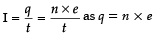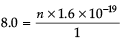⇒ n =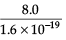= 5 x 1019 electrons per seconds.

Q.31: A wire has resistance 15 Ω. It is bent in the form of equilateral triangle. The effective resistance between any two vertices will be:
(a) 6.66 Ω
(b) 15 Ω
(c) 5 Ω
(d) 3.33 Ω

A wire of resistance 15 Ω bent into an equilateral triangle. As resistance is directly proportional to length.
So, resistance of each arm = 15/3 = 5Ω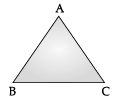Since, RAC and RBC are in series, their combine resistance = 5 + 5 = 10 Ω.
This 10 Ω resistance and RAB are in parallel. Therefore, equivalent resistance between A and B,
=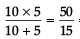= 3.33 Ω

Q.32: If a copper is shaped such that its resistance is minimum, then its length L and diameter d will b
(a) L, 3d
(b) L/3, 3d

(c) 3L, 3d
(d) L/3, d

Since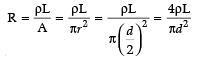Hence, R ∝ L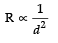Therefore, when length becomes L/3 and diameter becomes 3d. The new resistance is minimum among all the instances.

Q.33: Two bulb of 60 W and 80 W are connected to 220 V line. The ratio of their resistances:
(a) 4:3
(b) 3:4
(c) 16:9
(d) 9:16

Given that P1 = 60 W and P2 = 80 W and V = 220 V
Power is defined as P = V2/R
⇒ P ∝ 1 / R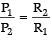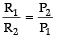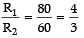Q.34: The current density (number of free electrons per m3) in metallic conductor is of the order of
(a) 1028
(b) 1023
(c) 1020
(d) 1015

The current density (number of free electrons per m3) in metallic conductor is of the order of 1028.

Q.35: What is the potential difference between points A and B in the  following circuit?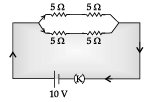(a) 10 V
(b) 5 V
(c) 2.5 V
(d) 20 V

Equivalent resistance of the circuit = ( 5 +5 ) P ( 5 +5 ) = 5Ω
Total circuit current = 10/5 = 2A
Current in each branch is 1 A
So, potential difference between points A and B i.e. across the 5 Ω resistor is 1 × 5 = 5 V.

Q.36: A solenoid of 1.5 metre length and 4.0 cm diameter has 10 turn per cm. A current of 5 A  is flowing through it. The magnetic field at axis inside the solenoid is
(a) 2π × 10–3 T
(b) 2π × 10–3 G
(c) 2π × 10–7 T
(d) 2π × 10–7

B = μ0ni = 4π x 10-7 x 5 x 10 102
= 2π x 10-3T

Q.37: A galvanometer of 100 Ω resistance gives full scale deflection for 10 mA current. To use it as an ammeter of 10 A range, the resistance of the shunt required is
(a) 10 Ω
(b) 0.10 Ω
(c) 0.01 Ω
(d) 0.001 Ω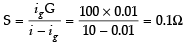Q.38: The magnetic flux linked with a coil is given by an equation ∅ = 5t2 + 2t + 3.
The induced e.m.f. in the coil at the 3 second will be
(a) 32 units
(b) 54 units
(c) 40 units
(d) 65 units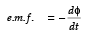=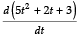= -(l0t + 2) = -32
e.m.f.t=3 = -(10 x 3 + 2) = -32

Q.39: The output of a step-down transformer is measured to be 24 V when connected to a 12 W Light bulb. The value of the peak current is
(a) 1√2A
(b) √2A
(c) 2A
(d) 2√2A

Given, Power associated with secondary,
Ps = 12 W
Secondary voltage, V= 24 V
Current in the secondary,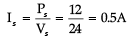Peak value of the current in the secondary,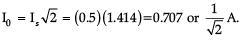Q.40: The heat produced in a given resistance in a given time by the sinusoidal current I0 sin wt will be the same as heat produced by a steady current of magnitude
(a) 0.707I0
(b) 1.412I0
(c) I0
(d) √I
0

Heat produced by AC is Heat produced by DC is I2R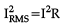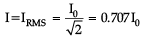Q.41: A coil having an area A0 is placed in a magnetic field which changes from B0 to 4B0 in time interval t. the e.m.f. induced in a coil will be
(a)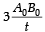(b)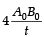(c)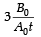(d)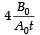Induced e.m.f.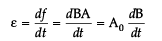=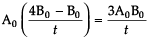Q.42: The time constant of an inductive coil is 2.0 × 10–3 second. When 60 Ω resistance is added in series, the time constant reduces to 9.5 × 10–3 second. If inductance of the coil is
(a) 20 mH
(b) 40 mH
(c) 50 mH
(d) 65 mH

Given,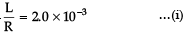And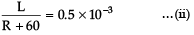Dividing eq (i) by eq (ii)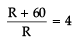⇒ R + 60 = 4R
⇒ 3R = 60
⇒ R = 60/3 = 20Ω
Substitute this value in (1)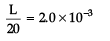⇒ L = 20 x 2.0 x 10-3
= 40 x 10-3
= 40mH

Q.43: A pure inductor of 15.0 mH is connected to a source of 220 V. Find the inductive reactance and rms current in the circuit if the frequency of the source is 50 Hz.
(a) 46.71 Ω
(b) 10.05 Ω
(c) 16.5 Ω
(d) 55.3 Ω

Given inductive reactance XL =2πfL
= 2 x 3.14 x 50 x 10-3
= 4.71Ω
The rms current in the circuit is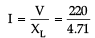= 46.71Ω

Q.44: A current of 2 A flows in a coil when connected to a 10 V dc source. If the same coil is connected to a 10 V, 50/π Hz ac source a current 1 A flows in the circuit. The inductance of the coil is
(a)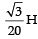(b)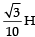(c)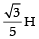(d)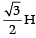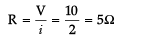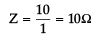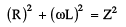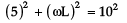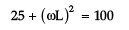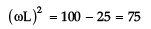⇒ ωL = √5/3                       -----(1)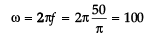⇒ 100L = √5/3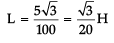Given below are two statements labelled as Assertion (A) and Reason (R)
Directions: In the following questions, a statement of Assertion (A) and is followed by a statement of Reason (R). Mark the correct choice as:
(a) Both Assertion (A) and Reason (R) are true, and Reason(R) is the correct explanation of (A).
(b)
Both Assertion (A) and Reason (R) are true, but Reason (R) is not the correct explanation of Assertion (A).
(c)
Assertion (A) is true, but Reason (R) is false.
(d)
Assertion (A) is false, but Reason (R) is true.
Q.45:
Assertion (A): Electric field is always normal to equipotential surfaces and along the direction of decreasing order of potential.
Reason (R): Negative gradient of electric potential is electric field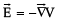So, the electric field is always perpendicular to equipotential surface. Negative gradient of electric potential is electric field. So, direction of electric field must be in the direction of the decreasing order of electric potential.

Q.46:
Assertion (A): Electrons are transferred from lower potential region to higher potential region.
Reason (R): Material which loses electron has positive charge and material which gains electrons has negative charge.

Electron has negative charged and moves towards the higher potential. By rubbing two materials, transferring of electron take place from the material in which electrons are less tightly bound to the other material in which electrons are more tightly bound. Material lost electrons becomes positively charged and materials gains electrons becomes negatively charged.
So, both assertion and reason are true. Reason is also the correct explanation of Assertion.

Q.47:
Assertion (A): Kirchhoff’s junction rule is applicable for any number of lines meeting at a point in an electrical circuit.
Reason (R): When there is a flow of steady current, then there is no accumulation of charge at the junction.

Kirchhoff’s junction rule is applicable at any point of an electrical circuit and there is no limitation of number of lines meeting at that point. So, the assertion is true.
While steady current is flowing there is no accumulation of charge at the junction. Total incoming charge = total outgoing charge. So, the reason is also true and explains the assertion.

Q.48:
Assertion (A): Magnetic field interacts with a moving charge only.
Reason (R): Moving charge produces a magnetic field.

Current carrying wire creates magnetic field. This magnetic field has no effect on a stationary charge. But when the charge also moves, it creates a current. This current produces a magnetic field. Two fields interact and the charge is deflected. So, the assertion is true. Moving charge creates a current which produces a magnetic field. So, the reason is also true. Reason is the correct explanation of A.

Q.49:
Assertion (A): An alternating current does not show any magnetic effect.
Reason (R): Alternating current changes direction with time.

Current or moving charged particle creates magnetic field irrespective of direct current or alternating current. So assertion is false. Alternating current changes direction with time. So, the reason is true, but cannot explain the assertion.

Section - C
This section consists of 6 multiple choice questions with an overall choice to attempt any 5. In case more than desirable number of questions are attempted, ONLY first 5 will be considered for evaluation.

Q.50: If a + Q charge is placed outside a closed surface. The net electric flux emerging out through the surface is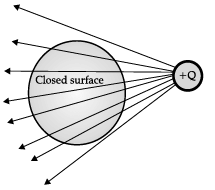(a) Q/2ε0
(b) 2Q/2ε0
(c) Q/ε0
(d) Zero

Gauss’s law states that the electric flux passing through any closed surface is 1/ε0  times of charge enclosed. Here, charge is outside the enclosed surface. Therefore, net electric flux emerging out through the surface is zero.
Another way of explaining is that the charge is placed outside the surface and the number of field lines entering the surface is equal to the number of field line going out. So, net flux through the close surface is zero.

Q.51: A circular loop of area 10 × 10–2 m2 rotates in a uniform magnetic field of 0.5 T. If the loop rotates about its diameter which is perpendicular to the magnetic field as shown in figure. The magnetic flux linked with the loop when its plane is normal to the field is
(a) 1 × 10–2 Wb
(b) 2 × 10–2 Wb
(c) 5 × 10–2 Wb
(d) zero

When magnetic flux is normal to the field, q = 0°. Therefore,
∅ = BAcosθ
= 0.5 x 10 x 10-2 x cos0o

CASE STUDY

The electric field is defined as the space surrounding by a charge in which all other test charge would experience force. It is a vector quantity that has both magnitude and direction. The magnitude of the vector is the electric field intensity, which is defined as the force per unit charge. Additionally, electric field strength is proportional to the density of lines of force i.e., electric field strength at a point is proportional to the number of lines of force cutting a unit area element placed normal to the field at that point. The direction of the field is considered to be the same as the direction of the force, and it is expected to exert on a positive test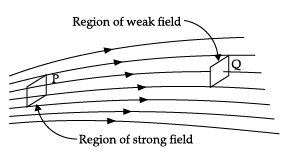charge. When the electric field is radially outward, then it is considered as a positive charge, and when the electric field is radially inward, then it is considered as a negative charge. The SI unit of the electric field is volt per meter.

Q.52: Electric lines of force of a negative point charge are
(c) parallel lines
(d) circular loops

The direction of the electrostatic force is outward from the positive charge and towards the negative charge. Hence, the electric field lines are inward for negative charge.
Additionally, for a point charge, electric lines of force are never circular, they are radial in nature.

Q.53: Which of the statement is false for electric lines of force?
(a) They start from a positive charge and terminate on a negative charge.
(b) They always form closed loops.
(c) They are parallel and equally spaced in uniform electric field.
(d) electric field line curves are continuous curve.

Since, electric field lines start from a positive charge and terminate on a negative charge. If field lines form closed loops, the origin points and termination points must be same which is not possible.

Q.54: Electric field lines are curved in case of
(a) single positive and negative charge
(b) two like charges
(c) two equal and opposite charges
(d) Both (B) and (C)

Field lines due to two like charges and two unlike charges having same magnitudes are shown in figure below. It is clear from the figure that field lines are the curved lines in these two cases, but do not form closed loops.
(a) Field lines due to two like charges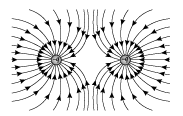(b) Field lines due to two unlike charges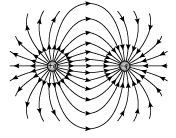Q.55: Electric field lines are ___________ to the surface charge.
(a) perpendicular
(b) parallel
(c) oblique
(d) None of these

If electric field line is not perpendicular to the surface, then it will have two components:
(1) electric field component parallel to the surface and
(2) electric field component perpendicular to the surface.
Where, perpendicular component will not affect the surface charge, whereas electric field parallel component will push the charges at rest into motion. This violet the approximation in electrostatics. Therefore, electric field cannot be any other direction. It has to be in perpendicular direction.

The document Class 12 Physics: CBSE Sample Question Paper- Term I (2021-22)- 4 Notes | Study Physics Class 12 - NEET is a part of the NEET Course Physics Class 12.
All you need of NEET at this link: NEETUse Code STAYHOME200 and get INR 200 additional OFF

## Physics Class 12

134 videos|388 docs|213 tests

### How to Prepare for NEET

Read our guide to prepare for NEET which is created by Toppers & the best Teachers

Track your progress, build streaks, highlight & save important lessons and more!

,

,

,

,

,

,

,

,

,

,

,

,

,

,

,

,

,

,

,

,

,

;